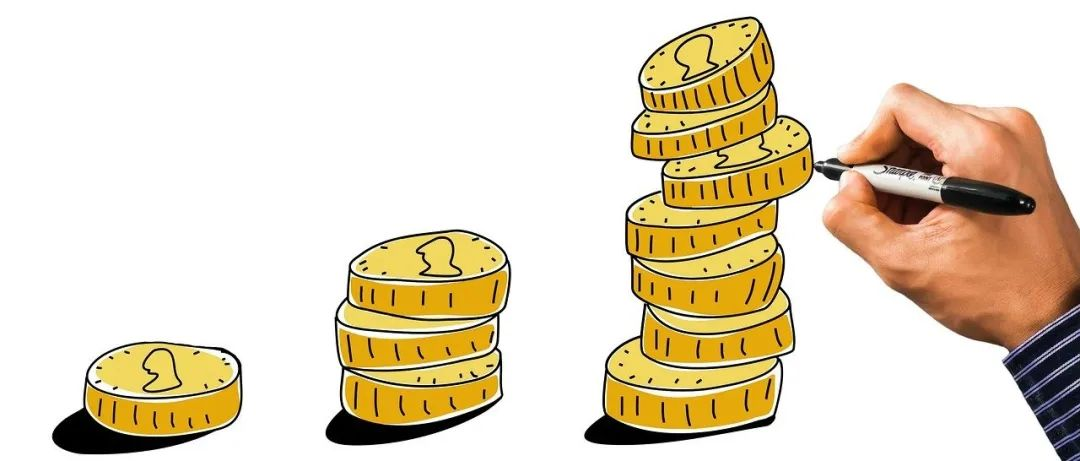## 年收入\$500和\$225,000的数据科学家之间有什么区别？

5个鲜为人知的 Python 库！帮你的下一个NLP项目起航

``````df = pd.read_csv("../input/kaggle-survey-2019/multiple_choice_responses.csv", low_memory = False)
df = df[~df['Q5'].isin(["Student", "Not employed"])]``````

``````cols = ['Q1', 'Q2', 'Q3', 'Q4', 'Q5', 'Q10']
plot_distirubution(df, cols)``````

``````df['compensation_num'] = df['Q10'].str.replace('\$', '')
df['compensation_num'] = df['compensation_num'].str.replace(',', '')
df['compensation_num'] = df['compensation_num'].str.replace('> 500000', '600000')

quenstion_dict = {}
for index, value in enumerate(df.loc[0, :]):
quenstion_dict[df.columns[index]] = value

df['low_compensation_num'] = df.loc[1:, 'compensation_num'].str.split('-').str
df['high_compensation_num'] = df.loc[1:, 'compensation_num'].str.split('-').str

df['low_compensation_num'] = df['low_compensation_num'].fillna(-1)
df['high_compensation_num'] = df['high_compensation_num'].fillna(-1)

df['low_compensation_num'] = df['low_compensation_num'].astype(int)
df['high_compensation_num'] = df['high_compensation_num'].astype(int)

df['compensation_num'] = (df['low_compensation_num'] + df['high_compensation_num']) / 2
df = df[df['compensation_num'] != -1]

df = df.drop(['low_compensation_num', 'high_compensation_num'], 1)
df['compensation_num_group'] = pd.qcut(df['compensation_num'], 3, labels=["low", "medium", "high"])``````

``````questions = group_cols(df)
score_cols = find_distribution_diff(df, questions, 'compensation_num_group')
sns.set(font_scale=1.2)
plot_salary(df, score_cols, quenstion_dict, target='compensation_num_group', country='all')``````
``plot_parallel_categories_salary(df, country='all')``
``plot_treemap_salary(df, target='compensation_num_group', country='all')``

``plot_point_salary(df, target='compensation_num_group', country='all')``